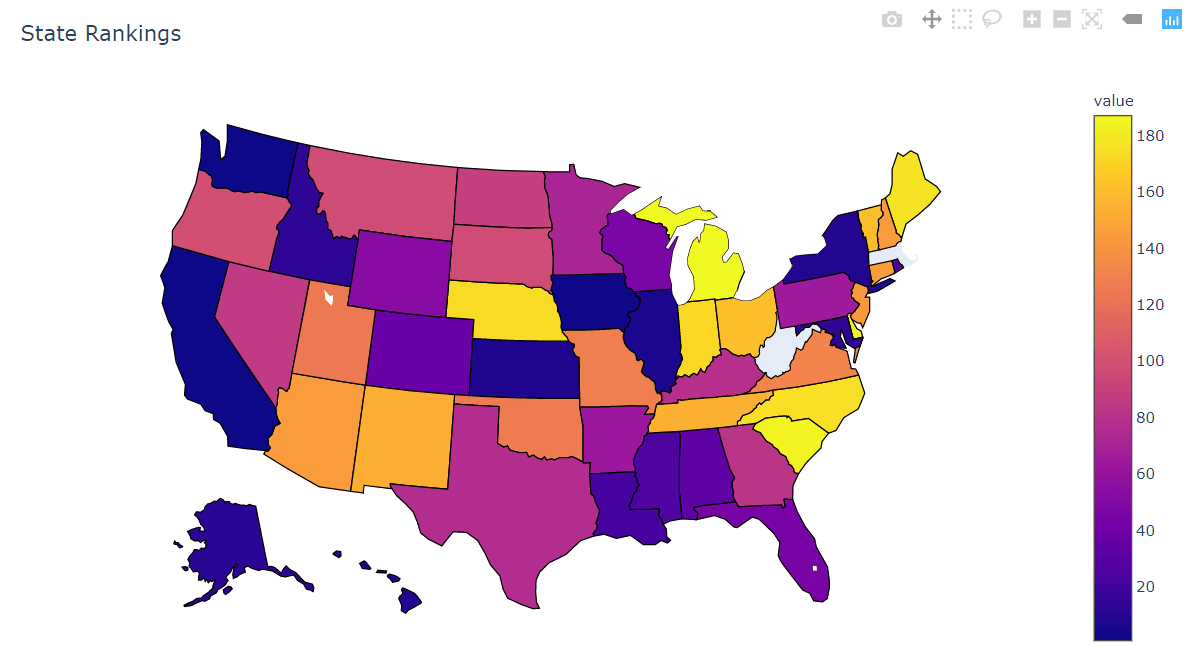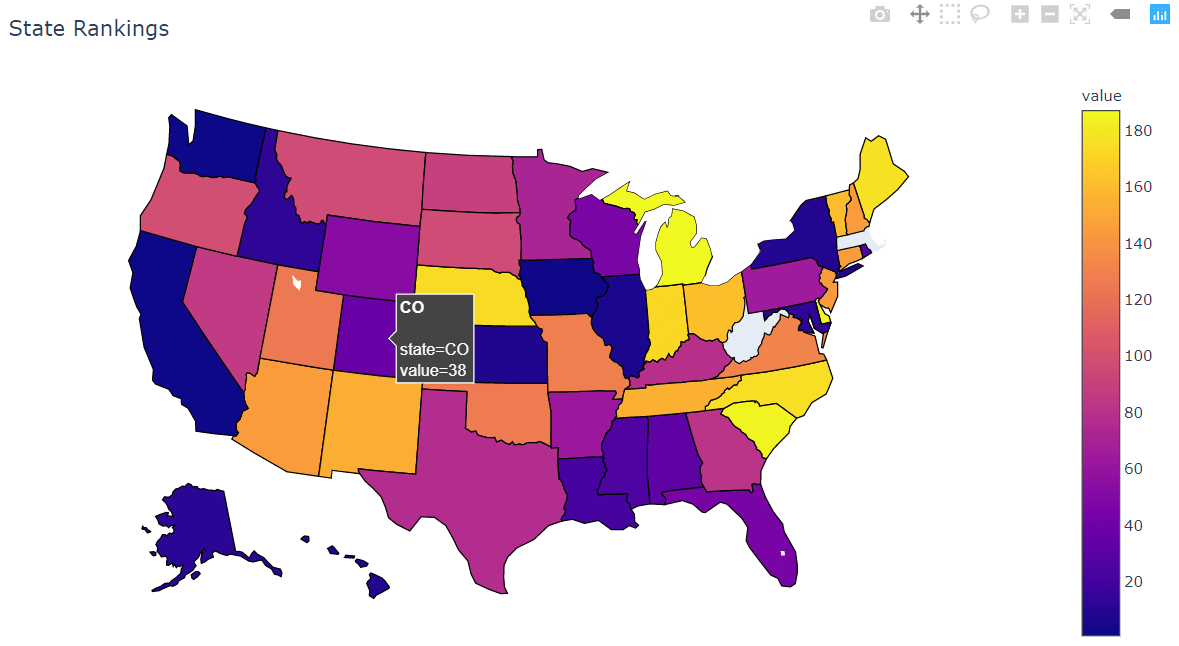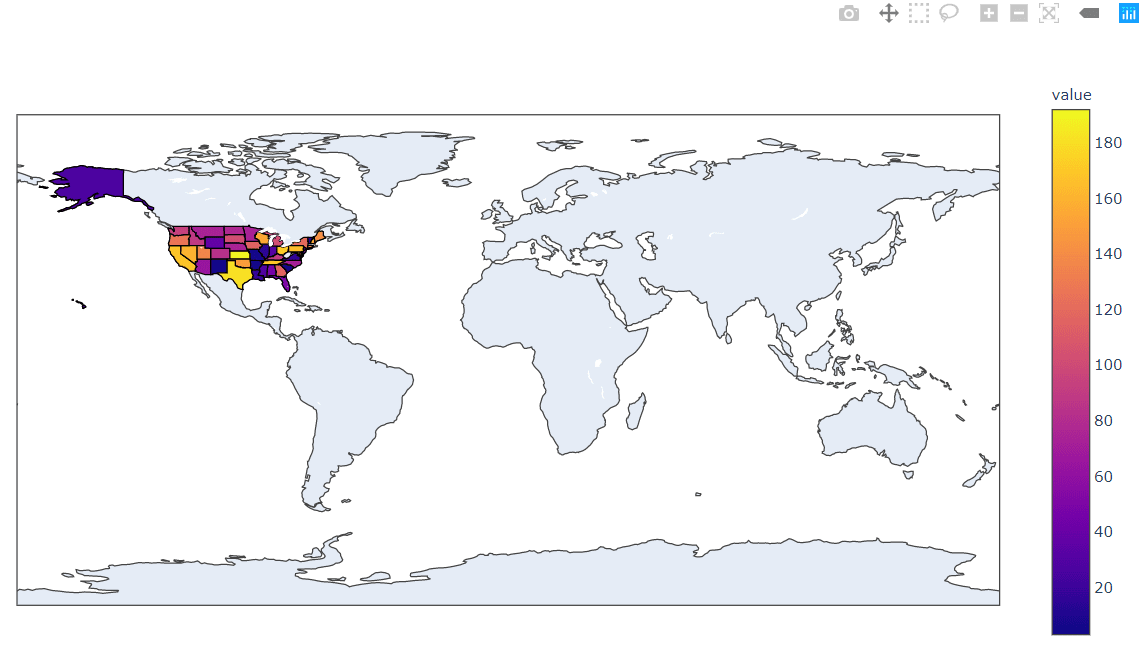## Quick Start: Creating a US State Choropleth Map with Python Plotly

A choropleth map is an interactive geographic color heatmap for plotting data on top of a region of geography. To create your own, first, ensure you have Plotly for Python installed:

To create a choropleth map of US states, first import your data into a Pandas DataFrame. The Pandas DataFrame should at a minimum have a “state” column containing the two-letter USPS State abbreviation values used to define data locations, and a “value” column containing the float values used to define a state’s color. Assuming the DataFrame is stored as `states_df`, the following code will produce a US State choropleth map:

``````import plotly.express as px  # Be sure to import express
fig = px.choropleth(state_df,  # Input Pandas DataFrame
locations="state",  # DataFrame column with locations
color="value",  # DataFrame column with color values
hover_name="state", # DataFrame column hover info
locationmode = 'USA-states') # Set to plot as US States
fig.update_layout(
title_text = 'State Rankings', # Create a Title
geo_scope='usa',  # Plot only the USA instead of globe
)
fig.show()  # Output the plot to the screen``````

When you run this Python Plotly code, you’ll get the following interactive plot:Notice that this is an interactive map, allowing for movement, zoom, and hover annotations:Can't get enough Python?

Python is powerful! Show me more free Python tips

## Introduction to US State Choropleths Maps with Plotly

Heatmap plots over geographic regions, or a choropleth in the formal definition, have been a useful tool for assessing the regional spread of data since the dawn of cartography. It continues to hold many uses today. Having to manually search for cartographic data sets and plot coordinate data with a litany of projection choices can be painful, but Plotly provides an easy-to-use Python package for plotting your data on a wide array of predefined maps.

This tutorial will focus on plotting data on a map of US States using a pre-made `state_df` Pandas DataFrame that has the following format:

``````state_df.info()
> <class 'pandas.core.frame.DataFrame'>
> RangeIndex: 49 entries, 0 to 48
> Data columns (total 2 columns):
> state    49 non-null object
> value    49 non-null int64
> dtypes: int64(1), object(1)
> memory usage: 864.0+ bytes

> 	state	value
> 0	   AL	   33
> 1	   AK	   12
> 2	   AZ	  144
> 3	   AR	   63
> 4	   CA	    1``````

Our DataFrame has a “state” column that contains the two-letter USPS State abbreviation corresponding to the state in which the data is represented. This format is crucial because Plotly will automatically associate each state abbreviation with its location on the map. The DataFrame also has a “value” column containing an arbitary set of float or integer values to be plotted with the associated state.

Note: Plotly expects DataFrames to be in the “tidy” format, or that each row contains a single record and each column contains a feature for that record. This means that each state should have at most one entry in the DataFrame.

## Using Plotly Python Modules

Now that we have our Pandas DataFrame created and in the proper format, we can use the tools provided by Plotly to create our choropleth map.

### Importing Plotly Express

First we will need to import the `plotly.express` module into our interpreter. It’s a common error to import this module as follows:

``````import plotly
x = plotly.express.[function](...)  # Not correct!``````

Importing this way will raise an import exception, as `plotly.express` is a separate Python package that is included with the Plotly installation. This is due to a historical quirk where old Plotly versions needed a dedicated installation of a `plotly_express` package that is now included in `plotly`. The correct way to import the module is as follows:

``````import plotly.express as px  # Here we've used px as a shortcut alias
x = px.[function](...)  # Correct!``````

Now we can use `px` as a shorthand form of calling the `plotly.express` module.

### Using the Plotly choropleth Function

Creating the choropleth map with Plotly in Python is extremely easy with the built-in `choropleth` function. Let’s look at the full function call first before we break down the arguments:

``````import plotly.express as px  # Be sure to import express
fig = px.choropleth(state_df,  # Input Pandas DataFrame
locations="state",  # DataFrame column with locations
color="value",  # DataFrame column with color values
hover_name="state", # DataFrame column hover info
locationmode = 'USA-states') # Set to plot as US States
fig.show()  # Output the plot to the screen``````
• `state_df`: The first argument specifies the Pandas DataFrame we created previously
• `locations="state"`: The `locations` argument specifies which column of the Pandas DataFrame contains the state abbreviations, which we’ve set to the state column for our particular DataFrame.
• `color="value"`: The `color` argument specifies which column of the Pandas DataFrame is used to color the given state region, which we’ve set to be the value column.
• `hover_name="state"`: The `hover_name` argument specifies which column of the Pandas DataFrame is used provide additional information to the user when the cursor is hovered over the region. We’ve set it to the state column to simply show the abbreviation of the state. You can get more creative in your own application.
• `locationmode = 'USA-states'`: The `locationmode` argument tells Plotly what kind of inputs it should expect to see in the `locations` column. We’ve set it to the builtin 'USA-states' option to treat the `locations` inputs as USA state identifiers.

Running the `choropleth` function to create a figure, and using the `fig.show()` command to render the figure onto our screen will present the following:Hmm, that’s certainly a choropleth map of the US States, but it includes the whole planet! Let’s see what we can do to simplify the plot.

Can't get enough Python?

Python is powerful! Show me more free Python tips

### Changing Choropleth Plotting Regions

We can change figure plotting parameters by calling the `update_layout` method on the created `fig` object. We can create a title for the figure using the `title_text` parameter, and we can tell Plotly to plot a USA-only version of the map using the option `geo_scope='usa'`. With this information, we can update our function call as follows:

``````import plotly.express as px  # Be sure to import express
fig = px.choropleth(state_df,  # Input Pandas DataFrame
locations="state",  # DataFrame column with locations
color="value",  # DataFrame column with color values
hover_name="state", # DataFrame column hover info
locationmode = 'USA-states') # Set to plot as US States
fig.update_layout(
title_text = 'State Rankings', # Create a Title
geo_scope='usa',  # Plot only the USA instead of globe
)
fig.show()  # Output the plot to the screen``````

Note: We called the `update_layout` method after the figure was created with the `choropleth` function but before we called the `fig.show()` method. This is an important ordering as the plot is “lost” after the `fig.show()` method is called.

Now we get the close-up geographical plot we wanted:Again, this is an interactive map so you can move it, zoom, and get hover annotations based on what we input to the `hover_name` option:I hope you enjoyed this tutorial on Python choropleth maps. Subscribe using the form below and share this article on Facebook and Twitter. When you spread the word on social media, you’re helping us grow so we can continue to provide free tutorials like this one.

Can't get enough Python?